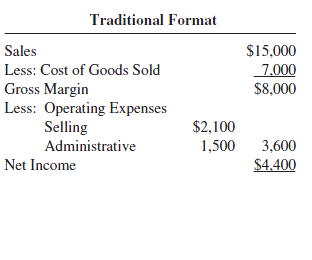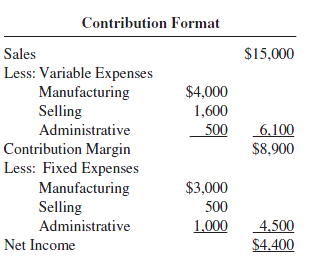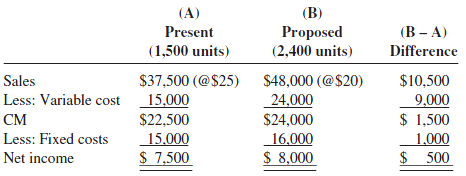Connect with us

Break Even Point and Contribution Margin AnalysisPublished

onBreak even point and contribution margin analysis, also known as cost-volume-profit (CVP) analysis, helps managers perform many useful analyses. It deals with how profit and costs change with a change in volume. More specifically, it looks at the effects on profits of changes in such factors as variable costs, fixed costs, selling prices, volume, and mix of products sold. By studying the relationships of costs, sales, and net income, management is better able to cope with many planning decisions. Break-even analysis determines the break-even sales. Break-even point—the financial crossover point when revenues exactly match costs—does not show up in corporate earnings reports, but managers find it an extremely useful measurement in a variety of ways.

Through this post, I am going to demonstrate various break even point and contribution margin analysis in details with load of case examples. It’s not merely about break even point and contribution margin formula and calculation, it is also about the concept and the tax effect. Enjoy!

Questions Answered by Break-even and Contribution Margin Analysis

Break-even and contribution margin analysis tries to answer these five questions:

• What sales volume is required to break even?
• What sales volume is necessary to earn a desired profit?
• What profit can be expected on a given sales volume?
• How would changes in selling price, variable costs, fixed costs, and output affect profits?
• How would a change in the mix of products sold affect the break-even and target income volume and profit potential?

Contribution Margin Income Statement

The traditional income statement for external reporting shows the functional classification of costs, that is, manufacturing costs versus non-manufacturing expenses (or operating expenses). An alternative format of income statement, known as the contribution margin income statement, organizes the costs by behavior rather than by function. It shows the relationship of variable costs and fixed costs a given cost item is associated with, regardless of the functions.

The contribution approach to income determination provides data that are useful for managerial planning and decision making. The statement highlights the concept of contribution margin, which is the difference between sales and variable costs. The traditional format emphasizes the concept of gross margin, which is the difference between sales and cost of goods sold.

These two concepts are independent and have nothing to do with each other. Gross margin is available to cover non-manufacturing expenses, whereas contribution margin is available to cover fixed costs. A comparison is made between the traditional format and the contribution format below:Contribution Margin [Concept—Formula—Case Examples]

For accurate break-even and contribution margin analysis, a distinction must be made between costs as being either variable or fixed. Mixed costs must be separated into their variable and fixed components.

In order to compute the break-even point and perform various break-even and contribution margin analyses, note these important concepts:

Contribution Margin (CM). The contribution margin is the excess of sales (S) over the variable costs (VC) of the product or service. It is the amount of money available to cover fixed costs (FC) and to generate profit. Symbolically, CM = S – VC.

Unit CM. The unit CM is the excess of the unit selling price (p) over the unit variable cost (v). Symbolically, unit CM = p – v.

CM Ratio. The CM ratio is the contribution margin as a percentage of sales, that is:

CM ratio = CM/S = [S-VC]/s = 1-[VC/S]

The CM ratio can also be computed using per-unit data:

CM ratio = Unit CM/p = [p-v]/p = 1-[v/p]

Note: that the CM ratio is 1 minus the variable cost ratio. For example, if variable costs account for 70 percent of the price, the CM ratio is 30 percent.

Example 1

To illustrate the various concepts of CM, consider these data:From the data listed, CM, unit CM, and the CM ratio are computed as:

CM = S – VC = \$37,500-\$15,000 = \$22,500
Unit CM = p-v = \$25 – \$10 = \$15
CM Ratio = CM/S= \$22,500/\$37,500 = 60% 0r Unit CM/p = \$15/\$25 = 0.6 = \$60%

Break-even Point Analysis

The break-even point represents the level of sales revenue that equals the total of the variable and fixed costs for a given volume of output at a particular capacity use rate. For example, one might want to ask the break-even occupancy rate (or vacancy rate) for a hotel or the break-even load rate for an airliner.

Generally, the lower the break-even point, the higher the profit and the less the operating risk, other things being equal. The break-even point also provides non-financial managers with insights into profit planning.

Break Even Point can be computed using these formulas:

Break-even point in units = Fixed Cost/Unit CM
Break-even point in dollars = Fixed Cost/CM Ratio

Using the same data given in Example 1, where unit CM = \$25 – \$10 = \$15 and CM ratio = 60%, we get:

Break-even point in units = \$15,000/\$15 = 1,000 units
Break-even point in dollars = \$15,000/0.6 = \$25,000

Or, alternatively:

1,000 units x \$25 = \$25,000

Determination of Target Income Volume

Besides determining the break-even point, break-even and contribution margin analysis determines the sales required to attain a particular income level or target net income.

The formula is:

Target Income Volume = [Fixed Cost + Target Income]/Unit CM

Example 3

Using the same data given in Example 1, assume that Lie Dharma Store wishes to attain a target income of \$15,000 before tax.

Then the target income volume would be:

(\$15,000 + \$15,000) / (\$25 – \$10) = \$30,000/\$15 = 2,000 units

Impact of Income Taxes

If target income is given on an after-tax basis, the target income volume formula becomes:

Target income volume = [Fixed costs + (Target after-tax income/(1-tax rate)]/Unit CM

Example 4

Assume in Example 1 that Lie Dharma Store wants to achieve an after-tax income of \$6,000. The tax rate is 40 percent. Then:
Target Income Volume
= [\$15,000 + (\$6,000/(1-0.4)]/\$15
= [\$15,000 + \$10,000]/15 = 1,667 units

Margin of Safety

The margin of safety is a measure of difference between the actual sales and the break-even sales. It is the amount by which sales revenue may drop before losses begin, and is expressed as a percentage of expected sales:

Margin of safety = Break-even sales / Expected sales

The margin of safety is used as a measure of operating risk. The larger the ratio, the safer the situation since there is less risk of reaching the break-even point.

Example 5

Assume Lie Dharma Store projects sales of \$35,000 with a break-even sales level of \$25,000. The projected margin of safety is:

[\$35,000 – \$25,000]/\$35,000 = \$28.57%

Applications of Contribution Margin Analysis and “What-If” Analysis

The concepts of contribution margin and the contribution income statement have many applications in profit planning and short-term decision making. Many “what-if” scenarios can be evaluated using them as planning tools, especially utilizing a spreadsheet program. Some applications are illustrated in Examples 6 to 10 using the same data as in Example 1.

Example 6

Recall from Example 1 that Lie Dharma Store has a CM of 60 percent and fixed costs of \$15,000 per period. Assume that the company expects sales to go up by \$10,000 for the next period.

How much will income increase?

Using the CM concepts, we can quickly compute the impact of a change in sales on profits. The formula for computing the impact is:

Change in net income = Dollar change in sales x CM ratio

Thus:

Increase in net income = \$10,000 x 60% = \$6,000

Therefore, the income will go up by \$6,000, assuming there is no change in fixed costs.
If we are given a change in unit sales instead of dollars, then the formula becomes:

Change in net income = Change in unit sales x Unit CM

Example 7

Assume that the store expects sales to go up by 400 units.

How much will income increase?

From Example 1, the company’s unit CM is \$15. Again, assuming there is no change in fixed costs, the income will increase by \$6,000:

400 units x \$15 = \$6,000

Example 8

What net income is expected on sales of \$47,500?

The answer is the difference between the CM and the fixed costs:

CM: \$47,500 x 60% = \$28,500
Less: Fixed costs      = \$15,000
Net income               = \$13,500

Example 9

Lie Dharma Store is considering increasing the advertising budget by \$5,000, which would increase sales revenue by \$8,000.

Should the advertising budget be increased?

The answer is no, since the increase in the CM is less than the increased cost:

Increase in CM: \$8,000 x 60%   = \$4,800
Decrease in net income             = \$(200)

Example 10

Consider the original data. Assume again that Lie Dharma Store is currently selling 1,500 units per period. In an effort to increase sales, management is considering cutting its unit price by \$5 and increasing the advertising budget by \$1,000. If these two steps are taken, management feels that unit sales will go up by 60 percent.

Should the two steps be taken?

The answer can be obtained by developing comparative income statements in a contribution format:Sales Mix Analysis

Break-even and cost-volume-profit analysis requires some additional computations and assumptions when a company produces and sells more than one product. In multi-product firms, sales mix is an important factor in calculating an overall company break-even point.

Different selling prices and different variable costs result in different unit CM and CM ratios. As a result, the break-even points and CVP relationships vary with the relative proportions of the products sold, called the sales mix.

In break-even and CVP analysis, it is necessary to predetermine the sales mix and then compute a weighted average unit CM. It is also necessary to assume that the sales mix does not change for a specified period.

The break-even formula for the company as a whole is:

Break-even sales in units (or in dollars)

= Fixed Costs / Weighted Average Unit CM [or CM Ratio]

Example 11

Assume that Putra, Inc., produces cutlery sets out of high-quality wood and steel. The company makes a deluxe cutlery set and a standard set that have these unit CM data:

Deluxe     Standard
Selling price                      \$15         \$10
Variable cost per unit          12          5
Unit CM                              \$3          \$5
Sales mix                            60%       40%
(based on sales volume)
Fixed costs                             \$76,000

The weighted average unit CM = (\$3)(0.6) + (\$5)(0.4) = \$3.80. Therefore, the company’s break-even point in units is:

\$76,000/\$3.80 = 20,000 units
which is divided in this way:

Deluxe: 20,000 units x 60%    =   12,000 units
Standard: 20,000 units x 40% =     8,000 units
20,000 units

Note: An alternative is to build a package containing 3 deluxe models and 2 standard models (3:2 ratio). By defining the product as a package, the multiple-product problem is converted into a single-product one. Then follow the next three steps:

Step 1. Compute the package CM

Deluxe     Standard
Selling price                  \$15            \$10
Variable cost per unit     12                5
Unit CM                          \$3              \$ 5
Sales mix                         3                 2
Package CM                   \$9             \$10
\$19 package total
\$76,000/\$19 per package = 4,000 packages

Step 2. Multiply this number by their respective mix units

Deluxe: 4,000 packages x 3 units     = 12,000 units
Economy: 4,000 packages x 2 units =    8,000 units
20,000 units

Example 12

Assume that Dharma, Inc., is a producer of recreational equipment. It expects to produce and sell three types of sleeping bags—the Economy, the Regular, and the Backpacker. Information on the bags follows:

The CM ratio for Dharma, Inc., is \$31,000/\$100,000 = 31%

Therefore, the break-even point in dollars is:

\$18,600/0.31 = \$60,000

which will be split in the mix ratio of 3:6:1 to give us these break-even points for the individual products:

Economy: \$60,000 x 30%         = \$18,000
Regular: \$60,000 x 60%           = \$36,000
Backpacker: \$60,000 x 10%     =     6,000
= \$60,000

One of the most important assumptions underlying CVP analysis in a multi-product firm is that the sales mix will not change during the planning period. But if the sales mix changes, the break-even point will also change.

Are you looking for easy accounting tutorial? Established since 2007, Accounting-Financial-Tax.com hosts more than 1300 articles (still growing), and has helped millions accounting student, teacher, junior accountants and small business owners, worldwide.